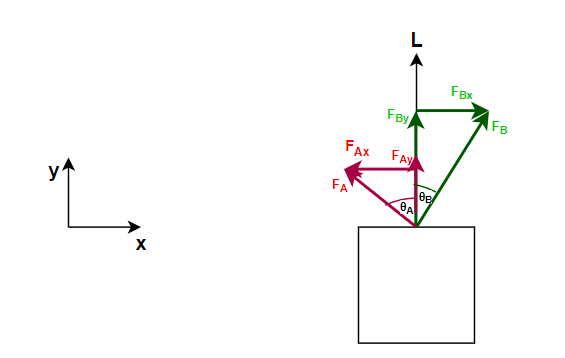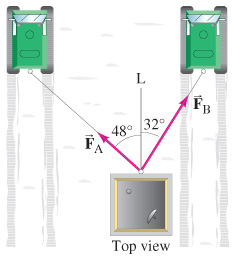# Problem: Two snowcats in Antarctica are towing a housing unit to a new location, as shown in the figure. The sum of the forces FA and FB exerted on the unit by the horizontal cables is parallel to the line L, and FA=4600 N .(a) Determine FB.(b) Determine the magnitude of FA + FB.

🤓 Based on our data, we think this question is relevant for Professor Kim, Westervelt, Witkov & Zengel's class at HARVARD.

###### FREE Expert Solution

We're asked to determine FB and the magnitude of the sum of the two forces.

This problem involves 2D Horizontal Forces. Like with any forces problems, we'll follow the same series of steps:

1. Draw a free body diagram (FBD), making sure to include and label coordinate axes
2. Set up Newton's Second Law equation ( ma )
3. Solve for the target variable

The equations to convert between components and magnitude-angle notation will always be useful when we're dealing with forces at different angles

Calculating components:

Looking at the nature of our problem, these equations will be:

This is because for the x-direction, we have opposite and hypotenuse and for y-direction, we have adjacent and hypotenuse to the angles.

Calculating magnitude:

$\overline{)\mathbf{|}\stackrel{\mathbf{⇀}}{\mathbit{F}}\mathbf{|}{\mathbf{=}}\sqrt{{{\mathbit{F}}_{\mathbit{x}}}^{\mathbf{2}}\mathbf{+}{{\mathbit{F}}_{\mathbit{y}}}^{\mathbf{2}}}}$

Step 1: Draw a free body diagram (FBD)

We'll simplify our problem by presenting it in an FBD.We'll use a standard coordinate system where forces to the right will be considered to be in positive x-direction and forces upwards will be in the positive y-direction

Remember, FAx is towards the left, hence negative (- FAx)###### Problem Details

Two snowcats in Antarctica are towing a housing unit to a new location, as shown in the figure. The sum of the forces FA and FB exerted on the unit by the horizontal cables is parallel to the line L, and FA=4600 N .

(a) Determine FB.
(b) Determine the magnitude of FA + FB.